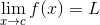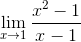### Day 02 - Finding Limits Numerically/Graphically - 08.18.15

Update
• Remind.com
• Text "@s1calc15" to 81010
• Calculator Cable
• What would you like to know about me?

Bell Ringer1. What does “lim” stand for in the notation above?

1. lim

2. limit

3. limerick

4. none of the above

2. What does f(x) stand for in the notation above?

1. an equation you are finding the limit of

2. a function

3. the limit

4. none of the above

3. What does x → c stand for?

1. It represents the value of x getting infinitesimally closer to c, but never actually reaching it.

2. It represent x being set equal to c.

3. It represent x turning into c.

4. none of the above

4. What does the L stand for?

1. It represents the value that the function is limited to.

2. It represents the limit of the function.

3. It represents the value of f(x) if x was getting infinitesimally closer to c.

4. none of the above

5. Find the limit for the following:1. undefined

2. 1

3. 2

4. 0

5. none of the above

Review

Lesson
• Limits
• How are limits found numerically and graphically? (checkpoints)

Exit Ticket
• Posted on the board at the end of the block.
Lesson Objectives
• How are limits found numerically and graphically?

#### In-Class Help Requests

Standard(s)
• APC.2
• Define and apply the properties of limits of functions.
• Limits will be evaluated graphically and algebraically.
• Includes:
• ​limits of a constant
• ​limits of a sum, product, and quotient
• ​one-sided limits
• ​limits at infinity, infinite limits, and non-existent limits*